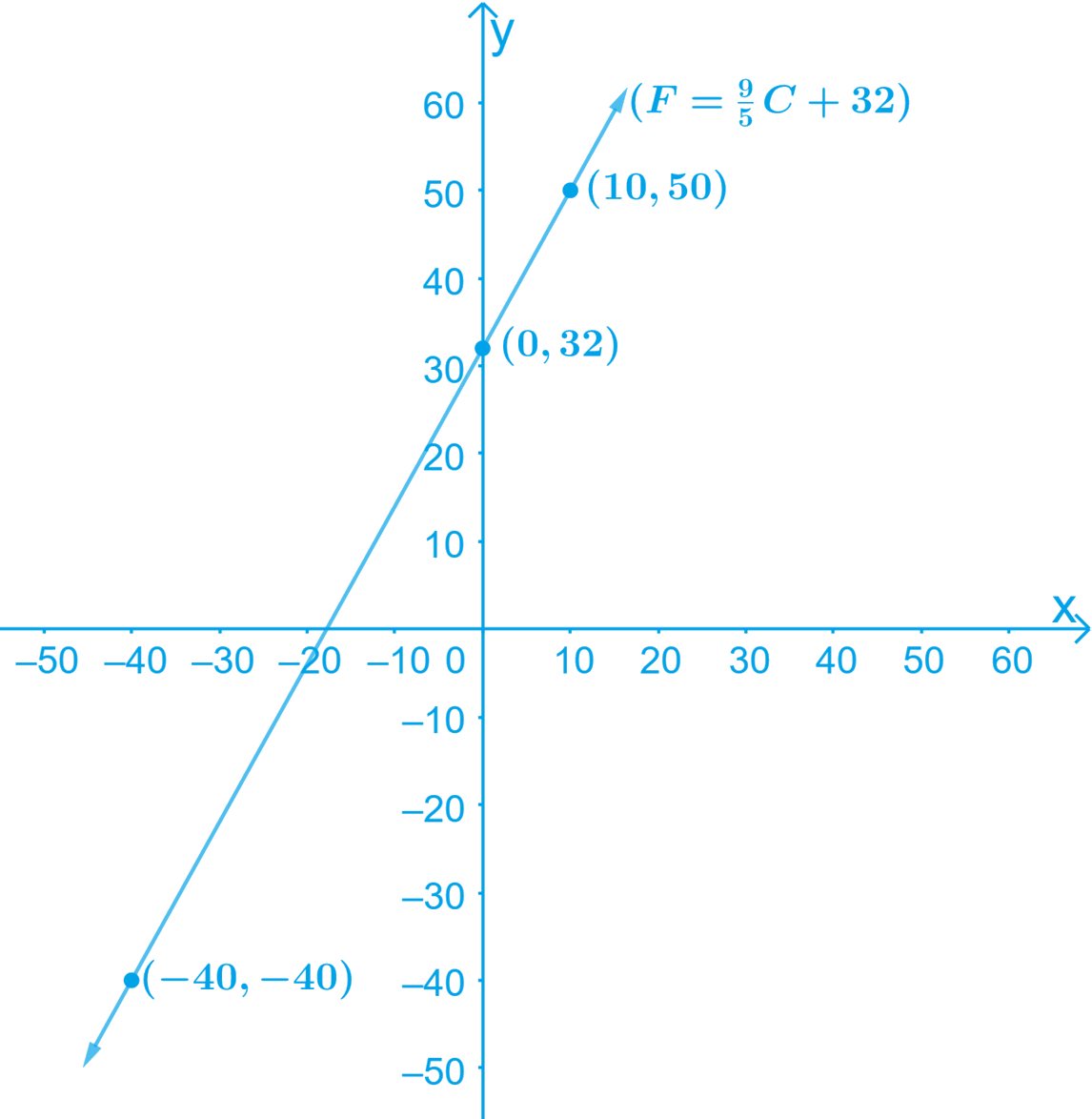# Ex.4.3 Q8 Linear Equations in Two Variables Solution - NCERT Maths Class 9

## Question

In countries like USA and Canada, the temperature is measured in Fahrenheit, whereas in countries like India, it is measured in Celsius. Here is a linear equation that converts Fahrenheit to Celsius: \begin{align} F = \left( \frac { 9 } { 5 } \right) C + 32 \end{align}

(i) Draw the graph of the linear equation above using Celsius for $$x$$-axis and Fahrenheit for $$y$$-axis.

(ii) If the temperature is $$30^{\circ} C$$, what is the temperature in Fahrenheit?

(iii) If the temperature is $$95^{\circ} F$$, what is the temperature in Celsius?

(iv) If the temperature is $$0^{\circ} C$$, what is the temperature in Fahrenheit and if the temperature is $$0^{\circ} F$$, what is the temperature in Celsius?

(v) Is there a temperature which is numerically the same in both Fahrenheit and Celsius? If yes, find it.

Video Solution
Linear Equations In Two Variables
Ex 4.3 | Question 8

## Text Solution

Steps:

(i) Given,

\begin{align} F = \left( \frac { 9 } { 5 } \right) C + 32 \end{align} \dots \text{ Equation } (1)

Equation (1) represents a linear equation of the form $$ax + by + c = 0$$ where $$C$$ and $$F$$ are the two variables.

By substituting different values for $$C$$ in the Equation (1), we obtain different values for $$F$$

When $$C = 0,$$

\begin{align}F = \left( {\frac{9}{5}} \right)C + 32 = \left( {\frac{9}{5}} \right)0 + 32 = 32\end{align}
When $$C = \,– 40,$$

\begin{align}F& = \left( {\frac{9}{5}} \right) \times ( - 40) + 32 \\&= - 72 + 32 = - 40\end{align}
When $$C = 10,$$

\begin{align}F = \left( {\frac{9}{5}} \right) \times (10) + 32 = 18 + 32 = 50\end{align}

Thus, we have the following table with all the obtained solutions:

 $$x$$ $$0$$ $$-40$$ $$10$$ $$y$$ $$32$$ $$-40$$ $$50$$

By Plotting the points $$(– 40, – 40)$$ and $$(10, 50)$$ on the graph point. On joining these points by line segment, we obtain the required graph

The graph of the line represented by the given equation as shown.(ii) Given:

Temperature $$= 30 ^\circ C$$

To find: $$F=?$$

We know that,\begin{align}F = \left( {\frac{9}{5}} \right)C + 32\end{align}

By Substituting the value of $$C= 30 ^\circ C$$ in the Equation above,

\begin{align}F &= \left( {\frac{9}{5}} \right)C + 32\\ &= \,\left( {\frac{9}{5}} \right)30 + 32\\ &= 54 + 32 \\&= 86 \end{align}

Therefore, the temperature in Fahrenheit is $$86^ {\circ} F$$.

(iii) Given:

Temperature $$=$$ $$95^ {\circ} F$$

To find: $$C= ?$$

We know that,\begin{align} F = \left( \frac { 9 } { 5 } \right) C + 32 \end{align}

By Substituting the value of temperature in the Equation above,

\begin{align}95 &= \left( {\frac{9}{5}} \right)C + 32\\95 - 32 &= \left( {\frac{9}{5}} \right)C\\63 &= \left( {\frac{9}{5}} \right)C\\\therefore C &= \frac{{63 \times 5}}{9} = 35\end{align}

Therefore, the temperature in Celsius is $$35^ {\circ} C$$.

(iv) We know that, \begin{align} F = \left( \frac { 9 } { 5 } \right) C + 32 \end{align}

If $$C = 0^ {\circ}C$$, then by Substituting the value in the above Equation,

\begin{align}F &= \left( {\frac{9}{5}} \right)0 + 32\\F &= 0 + 32\\F &= 32\end{align}

Therefore, if $$C = 0^ {\circ}C$$, then $$F = 32^ {\circ}F$$.

If $$F = 0^ {\circ}F$$, then by Substituting the value in the above Equation,

\begin{align}0 &= \left( {\frac{9}{5}} \right)C + 32\\\left( {\frac{9}{5}} \right)C &= - 32\\C &= \frac{{ - 32 \times 5}}{9} = - 17.77\end{align}

Therefore, if $$F = 0^ {\circ}F$$, then $$C = 17.8^ {\circ}C$$.

(v) We know that,

\begin{align} F = \left( \frac { 9 } { 5 } \right) C + 32 \end{align}

Let us consider, $$F = C$$

By Substituting this value in the Equation above,

\begin{align}&F = \left( {\frac{9}{5}} \right)F + 32\\&\left( {\frac{9}{5} - 1} \right)F + 32 = 0\\&\left( {\frac{4}{5}} \right)F = - 32\\&F = \frac{{ - 32 \times 5}}{4}\end{align}

Hence, $$F = \,–40^ {\circ}F$$

Yes, there is a temperature, $$−40^ {\circ}F$$, which is numerically the same in both Fahrenheit and Celsius.

Video Solution
Linear Equations In Two Variables
Ex 4.3 | Question 8
Learn from the best math teachers and top your exams

• Live one on one classroom and doubt clearing
• Practice worksheets in and after class for conceptual clarity
• Personalized curriculum to keep up with school